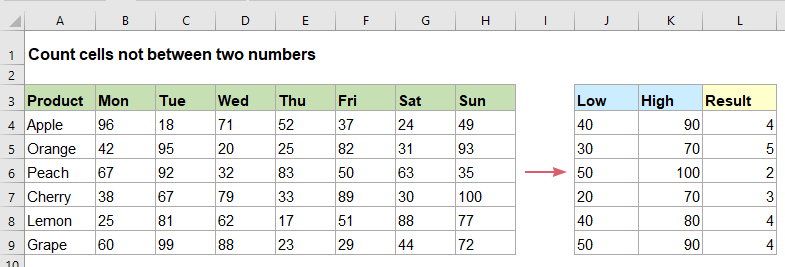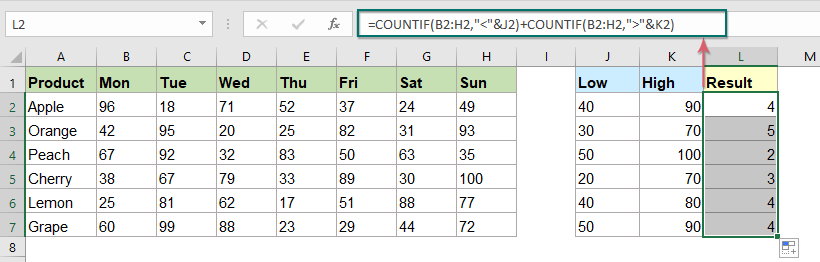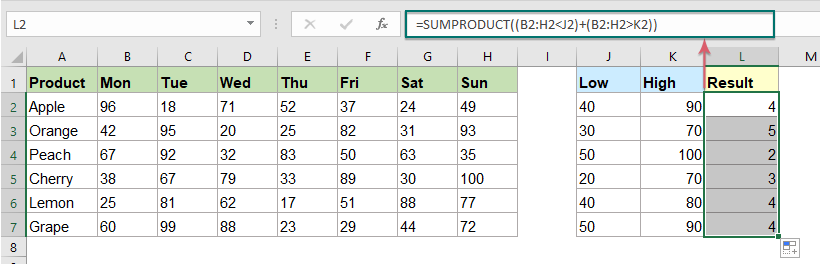## Count number of cells not between two given numbers in Excel

To count the number of cells between two numbers is a common task for us in Excel, but, in certain case, you may want to count the cells not between two given numbers. For example, I have a product list with the sales from Monday to Sunday, now, I need to get the number of cells which not between the specific low and high numbers as below screenshot shown. This article, I will introduce some formulas for dealing with this task in Excel.#### Count number of cells not between two given numbers with COUNTIF function

The COUNTIF function in Excel may help you to solve this job, the generic syntax is:

=COUNTIF(range,"<"&low)+COUNTIF(range,">"&high)
• range: The data range that contains the values you want to count;
• low: The cell containing the lower limit of the interval;
• high: The cell containing the higher limit of the interval;
• &: This separator is used to concatenate the cell value with the conditions.

1. Please enter or copy the following formula into a blank cell:

=COUNTIF(B2:H2,"<"&J2)+COUNTIF(B2:H2,">"&K2)

2. Drag the fill handle down to the cells that you want to apply this formula, then all the number of the cells which are not between the two given numbers have been calculated, see screenshot:##### Explanation of the formula:
• COUNTIF(B2:H2,"<"&J2): This COUNTIF function will get the number of cells below the value in J2, it returns the result:3.
• COUNTIF(B2:H2,">"&K2): This COUNTIF function will get the number of cells above the value in K2, it returns the result:1.
• COUNTIF(B2:H2,"<"&J2)+COUNTIF(B2:H2,">"&K2)=3+1: Add these two results to get the required logic: less than J2 or greater than K2.

#### Count number of cells not between two given numbers with SUMPRODUCT function

Here is also a SUMPRODUCT function which can help you to count the number of cells not between two numbers, the generic syntax is:

=SUMPRODUCT((range<low)+(range>high))
• range: The data range that contains the values you want to count;
• low: The cell containing the lower limit of the interval;
• high: The cell containing the higher limit of the interval;

1. Enter or copy the below formula into a blank cell:

=SUMPRODUCT((B2:H2<J2)+(B2:H2>K2))

2. And then, drag the fill handle down to the cells that you want to apply this formula, and you will get the result at once, see screenshot:##### Explanation of the formula:
• In this formula, you don't need to use & separator to concatenate the cell references, and the addition (+) sign here creates an OR logic.
• This formula will evaluate each array and return TRUE or FALSE for each value, as this: =SUMPRODUCT({FALSE,TRUE,FALSE,FALSE,TRUE,TRUE,FALSE}+{TRUE,FALSE,FALSE,FALSE,FALSE,FALSE,FALSE})
• And the math operation will convert the TRUE and FALSE values to 1s and 0s as this: =SUMPRODUCT({0,1,0,0,1,1,0}+{1,0,0,0,0,0,0})
• At last, add the two arrays to get the result: =SUMPRODUCT({1, 1, 0, 0, 1,1.0})=4.

#### Relative function used:

• COUNTIF:
• The COUNTIF function is a statistical function in Excel which is used to count the number of cells that meet a criterion.
• SUMPRODUCT:
• The SUMPRODUCT function can be used to multiply two or more columns or arrays together, and then get the sum of products.

#### More articles:

• Count Number Of Cells Not Equal To Many Values In Excel
• In Excel, you may easily get the number of cells not equal to a specific value by using the COUNTIF function, but have you ever tried to count the cells which are not equal to many values? For example, I want to get the total number of the products in column A but exclude the specific items in C4:C6 as below screenshot shown. This article, I will introduce some formulas for solving this job in Excel.
• Count Number Of Cells Equals To One Of Many Values In Excel
• Supposing, I have a list of products in column A, now, I want to get the total number of specific products Apple, Grape and Lemon which listed in range C4:C6 from column A as below screenshot shown. Normally, in Excel, the simple COUNTIF and COUNTIFS functions will not work in this scenario. This article, I will talk about how to solve this job quickly and easily with the combination of SUMPRODUCT and COUNTIF functions.

### The Best Office Productivity Tools

#### Kutools for Excel - Helps You To Stand Out From Crowd

 Popular Features: Find/Highlight/Identify Duplicates  |  Delete Blank Rows  |  Combine Columns or Cells without Losing Data  |  Round without Formula ... Super VLookup: Multiple Criteria  |  Multiple Value   |  Across Multi-Sheets  |  Fuzzy Lookup... Adv. Drop-down List: Easy Drop Down List  |  Dependent Drop Down List  |  Multi-select Drop Down List... Column Manager: Add a Specific Number of Columns  |  Move Columns  |  Unhide Columns  |  Compare Columns to Select Same & Different Cells ... Featured Features: Grid Focus  |  Design View  |  Big Formula Bar  |  Workbook & Sheet Manager  |  Resource Library (Auto Text)  |  Date Picker  |  Combine Worksheets  |  Encrypt/Decrypt Cells  |  Send Emails by List  |  Super Filter  |  Special Filter (filter bold/italic/strikethrough...) ... Top 15 Toolsets:  12 Text Tools (Add Text, Remove Characters ...)  |  50+ Chart Types (Gantt Chart ...)  |  40+ Practical Formulas (Calculate age based on birthday ...)  |  19 Insertion Tools (Insert QR Code, Insert Picture from Path ...)  |  12 Conversion Tools (Numbers to Words, Currency Conversion ...)  |  7 Merge & Split Tools (Advanced Combine Rows, Split Cells ...)  |  ... and more

Kutools for Excel Boasts Over 300 Features, Ensuring That What You Need is Just A Click Away...

Supports Office/Excel 2007-2021 and 365   |   Available in 44 Languages   |   Easy to Uninstall Completely#### Office Tab - Enable Tabbed Reading and Editing in Microsoft Office (include Excel)

• One second to switch between dozens of open documents!
• Reduce hundreds of mouse clicks for you every day, say goodbye to mouse hand.
• Increases your productivity by 50% when viewing and editing multiple documents.
• Brings Efficient Tabs to Office (include Excel), Just Like Chrome, Edge and Firefox.